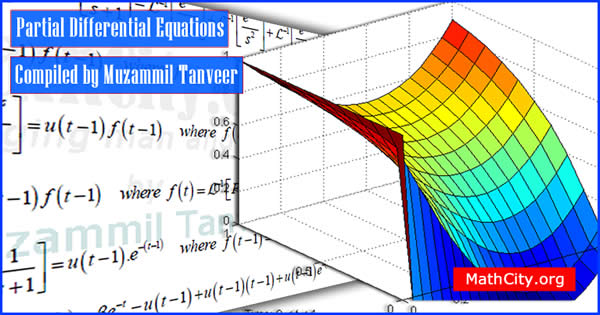# Partial Differential Equations by Muzammil TanveerThese notes are provided and composed by Mr. Muzammil Tanveer. We are really very thankful to him for providing these notes and appreciates his effort to publish these notes on MathCity.org

 Name Partial Differential Equations or PDEs Mr. Muzammil Tanveer 173 pages PDF (see Software section for PDF Reader) 1.21MB
• Differential Equations
• Types of Differential Equations
• Ordinary Differential Equations
• Partial Differential Equations
• Method of separation of variable or product method or Fourier method
• Some Eigen values and Eigen function
• Solution of Non-Homogeneous Equation
• Solution of Homogeneous Equation
• Laplace Equation
• Polar form of Laplace Equation
• Canonical form/ Normal form / Standard form
• General Transform
• Jacobian Transformation
• Canonical form of Hyperbolic equation
• Canonical form or Normal form of Parabolic equation
• Canonical form of Elliptic equation
• Gamma Function
• Piecewise Continuous function
• Laplace Transform
• Linearity Property
• First Shifting property
• Second Shifting property
• Inverse Laplace transform
• Convolution for Laplace transformation
• Convolution Theorem
• Fourier Transform
• Fourier Transform of Gaussian function
• Contour Integration
• Attenuation property
• Fourier Transformation derivatives of a function
• Fourier Sine and Cosine Transform
• Fourier Sine and Cosine Transform of derivatives
• Convolution form Fourier Transform

Please click on View Online to see inside the PDF.

• notes/partial-differential-equations-muzammil-tanveer